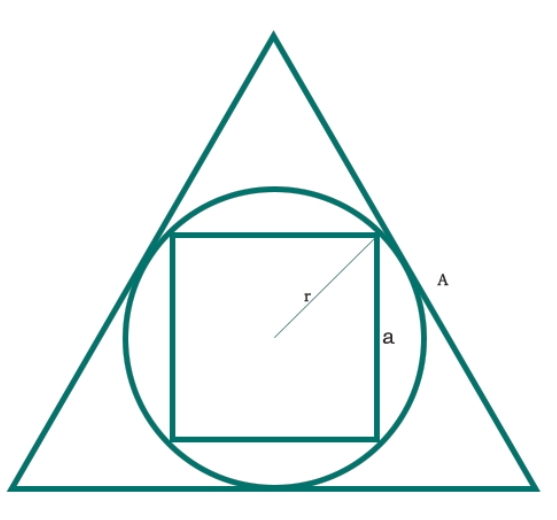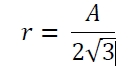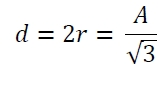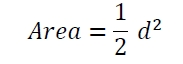# Area of a square inscribed in a circle which is inscribed in an equilateral triangle in C Program?

Here we will see the area of a square which in inscribed in one circle and that circle is inscribed in an equilateral triangle. The side of the square is ‘a’. The radius of the circle is ‘r’, and the side of the hexagon is ‘A’. The diagram will be like below.We know that the radius of a circle inscribed in a equilateral triangle is the inradius of the triangle. So the value is −So the diagonal of the square is −So the area of the square is −## Example

#include <iostream>
#include <cmath>
using namespace std;
float area(float A) { //A is the side of the triangle
if (A < 0) //if the value is negative it is invalid
return -1;
float d = A / sqrt(3);
float area = 0.5*d*d;
return area;
}
int main() {
float side = 10;
cout << "Area is: " << area(side);
}

## Output

Area is: 16.6667